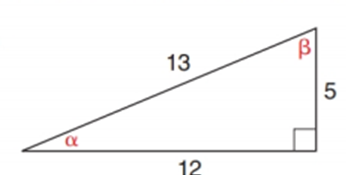Chapter 11.2, Problem 8E### Elementary Geometry for College St...

6th Edition
Daniel C. Alexander + 1 other
ISBN: 9781285195698

#### Solutions

Chapter
Section### Elementary Geometry for College St...

6th Edition
Daniel C. Alexander + 1 other
ISBN: 9781285195698
Textbook Problem
1 views

# Using the right triangle from Exercise 1, show that sin 2 α + cos 2 α = 1 Exercises 1.To determine

To find:

To show that sin2α+cos2α=1.

Explanation

Consider the following figure,

General formula for cosine and sine ratio is given below,

From the given figure 13 is the hypotenuse value since it is opposite to right angle.

Then 12 is the length of the leg adjacent to α and 5 is the length of leg opposite to α.

Therefore,

cosα=1213 and sinα=513

Consider sin2α+cos2α=1.

Substitute the values of cosα=1213 and sinα=513 in the above equation to get the following,

### Still sussing out bartleby?

Check out a sample textbook solution.

See a sample solution

#### The Solution to Your Study Problems

Bartleby provides explanations to thousands of textbook problems written by our experts, many with advanced degrees!

Get Started

#### Find more solutions based on key concepts